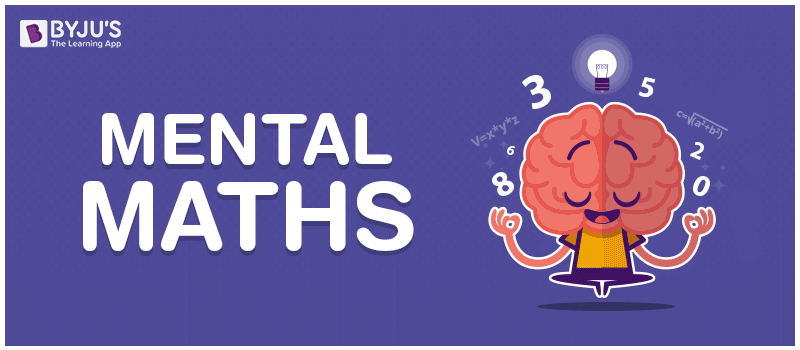Checkout JEE MAINS 2022 Question Paper Analysis : Checkout JEE MAINS 2022 Question Paper Analysis :

# Mental Maths For Class 2

Maths is one subject that is a must for any individual to understand the working of basic things in day-to-day life —as such, developing and sharpening Math skills is quite important even though some are terrified in dealing with the subject. That being said, learning maths in class 2 can be fun and simple, especially if we develop a keen interest in the topics, sharpen mental maths for class 2, and work towards solving the problems related to the subject early on.However, developing Math fluency isn’t always a smooth run, and it does take time and effort. But again, there are some shortcuts that can help students learn the subject and understand the concepts more easily. Mental Maths is one such shortcut. Besides, developing this skill is not as difficult as it sounds. With mental Maths, class 2 students can learn to make calculations easily and effectively using nothing but their brains. The only thing is they just need to practice.

There are several ways to add mental maths practice into everyday activities. For example, counting change, math games, keeping a score in scrabble and others. Incorporating exercises that involve using maths operations like addition, subtraction, multiplication, etc., will be helpful.

Meanwhile, mental maths also helps improve calibre and the brains activity that ultimately helps students solve problems quicker. Mental maths for class 2 CBSE helps to improve the learning capabilities of a student with quick and easy problems and exercises. Working on mental maths questions for class 2 will help students to solve questions during exams and also help them build a strong foundation for the coming years.

Check our maths worksheet for grade 2 so that the children can acquire efficient problem-solving skills that will be helpful in exam situations. In addition, you can also use the maths exercises that we are providing to help the kids practise the mental maths sections. It will help them to become familiar with tests that are usually administered in schools. The question papers come with instructions and guides.

## Class 2 Mental Maths Exercise:

 1. How many 10’s make 100? 2. What is 10 x 12 3. The time is 11:30. What was the time 2 hours ago? 4. How many days are there in 3 weeks? 5. What is 5 more than 27? 6. What comes next? 35, 34, 33, 32, 31, ___ 7. Double 25 8. 20 people are on a train. 5 more get in and 2 get down? How many people are there on the train? 9. I have Rs. 100. I buy candy for Rs 20 and a toy for Rs 50. How much money is left? 10. The temperature is 26 degrees. It gets 7 degrees hotter. What is the temperature now?

For other Maths-related content for class 2 and more, you can explore BYJU’S as we offer the latest syllabus, question papers, and sample papers.

## Frequently Asked Questions – FAQs

### What is the predecessor of 4?

The predecessor of 4 is 3.
Predecessor means subtract 1 from the given number i.e., 4-1=3

### What is the successor of 4?

The successor of 4 is 5.
Successor means add 1 to the given number i.e., 4+1=5

### What are values between 4 and 8?

The values between 4 and 8 are 5, 6, 7

### What will be the next number of sequence 28, 29, 30, 31,…?

The next number of the sequence is 32

### What is 3 more than 25?

3 more than 25 is 25+3 = 28.